Wednesday 17th August 2022
 All India Engineering / Architecture Entrance Examination (AIEEE) CBSE Guess > AIEEE > AIEEE Papers > 2009 > Chemistry AIEEE 2009 Chemistry Q. 1. Arrange the carbanions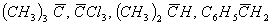, in order of their decreasing stability: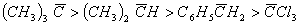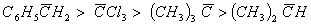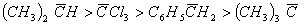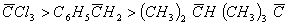Sol: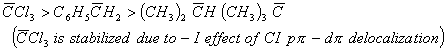Answer : (4) Q. 2. In Cannizzaro reaction given below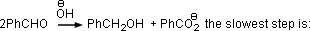the deprotonation of PhCH2OH the attack of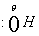at the carboxyl group the transfer of hydride of the carbonyl group the abstraction of proton from the carboxylic group Sol.: Cannizzaro reaction involves hydride transfer as the rate limiting step. Answer : (3) Q. 3. Two liquids X and Y form an ideal solution. At 300 K, vapour pressure of the solution containing 1 mol of X and 3 mol of Y is 550 mmHg. At the same temperature, if 1 mol of Y is further added to this solution, vapour pressure of the solution increases by 10 mmHg. Vapour pressure (in mmHg) of X and Y in their pure states will be, respectively: 500 and 600 200 and 300 300 and 400 400 and 600 Sol: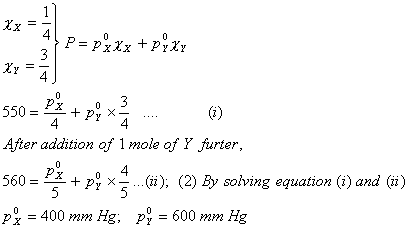Answer : (4) Q. 4. In a fuel cell, methanol is used as fuel and oxygen gas is used as an oxidizer. The reaction is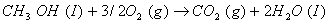; At 298 K standard Gibb’s energies of formation for CH3OH(l), H2O(l) and CO2(g) are –166.2, –237.2 and –394.4 kJ mol–1 respectively. If standard enthalpy of combustion of methanol is –726 kJ mol–1, efficiency of the fuel cell will be 97% 80% 87% 90% Sol: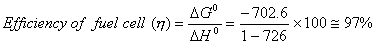Answer : (1)